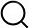# Also in the Article

Behavior of random genetic (RG) model

Constructing graphs from genetic encodings
Sci Rep, Jun 24, 2021;

Procedure

In this section we propose approximations for the transitions from subcritical to supercritical to connected phases of the RG model. We consider the critical point of the system to be equivalent to an ER graph’s critical point, when $⟨k⟩=1$. The density of the system was derived in-text, and multiplying by the number of nodes we can write:

where $π=2x-b$. We found it useful to solve for $Rcrit(x,b)$ which is the number of rules introduced to reach the critical point for a given x and b:

Alternatively, we consider the transition from supercritical to connected regime to occur when the nodes are ‘covered’ by wiring rules, by which we mean that there are sufficient rules for every node to participate in at least 1 rule if there were no overlap. In this manner, $Rcrit(x,b)$ can be found as the number of nodes divided by the number of nodes in a wiring rule:

Due to the cost of computation, the in-text results for RG networks were shown for b = 11 (Fig. (Fig.4,4, although Fig. Fig.3c3c is b = 12). Introducing many wiring rules and measuring the resulting network properties for larger systems, especially with statistically sufficient repeats, is not computationally feasible. As an indication that our results should be consistent for larger systems, we examine the scaling of density, LCC, and $#CC$ with system size (Fig. S6). We offer exemplary plots (Fig. S6) close to the three r values in Fig. Fig.4d–f4d–f (r = 15, 30, and 70) as well as for r = 160, which is the largest simulated system in Fig. Fig.44a–c.

Although we derived an equation for RG network density in-text, the $1-(1-π2)2r$ formulation does not account for saturation. We thus reframe density as the number of unique links selected from the set of all possible, under replacement. This can be expressed as:

where $n=2b-x-1∗(2b-x-1).$ We can see that density can be interpreted as a function of $b-x$, thus is determined by the relative size of x compared to b, rather than the system size itself. This observation is confirmed by numerical simulations: the density of the system should follow a similar pattern for larger systems (Fig. S6, left column).

The second network metric of interest to the RG model was LCC. We can approximate the size of the LCC by calculating the probability that a node was chosen by a wiring rule. This approximation assumes that a node, if chosen, will belong to the LCC, which we found to be generally valid for the supercritical regime (Fig. (Fig.4c).4c). We can express the relative size of the LCC as a problem of choosing unique nodes with replacement, allowing us to write:

where $n=2b-x.$ We again find that this is dependent on $b-x$, and find that systems of multiple sizes conform to the approximation (Fig. S6, middle column). Deviations from the analytical approximation can be attributed to the finite size: in the subcritical regime $LCC∼22x$, thus $LCC/N∼22x-b$, from which $x-b$ is no longer cleanly separable.

The final network metric considered was the number of connected components ($#CC$). This can be seen as the inverse of the LCC: $#CC$ is the number of nodes not selected by a rule. Thus, we express the relative $#CC$ as:

where $n=2b-x$ (Fig. S6, right column). This again can be parameterized in terms of b-x, except in the connected regime, where $#CC→1$ , thus $#CC/N→2-b$. Yet, if we think of LCC and $#CC$ as complementary measures, we then have approximates for all three documented regimes.

An important limit considered in graph models is the scaling of network metrics with system size, when a constant average degree is maintained. Specifically, how does the number of rules r(N) scale with system size $N=2b$ given a hand-imposed scaling of the average degree $⟨k⟩$?

Solving for the number of rules given the network density $ρ=1-1-22(x-b)2r$ and average degree $⟨k⟩=ρN$ gives

In the limit of $b>>1$, using $log(1+a)≈a$, () is simplified to

If, in addition, $b-x=g$ is constant, then the number of rules scales as

In this limit, therefore, the number of rules scales inversely with system size. This can be understood based on the fact that wiring rule sizes, in terms of the number of new edges they introduce, grow proportionally to the square of the system size N.

If, on the other hand, $b-x>>1$ (such as when x/b or x are kept constant), then

In this regime, the number of rules always scales as the system size N.

Q&A